• 双边滤波器
2020-10-07 17:58:59

双边滤波（Bilateral filter）是一种可以保边去噪的滤波器。之所以可以达到此去噪效果，是因为滤波器是由两个函数构成。

一个函数是由几何空间距离决定滤波器系数。另一个由像素差值决定滤波器系数。使用二维高斯函数生成距离模板，使用一维高斯函数生成像素差值模板。

对比两个filter：高斯低通滤波器和α-截尾均值滤波器（去掉百分率为α的最小值和最大之后剩下像素的均值作为滤波器）, 双边滤波器同时考虑了空间域与值域的差别，而Gaussian Filter和α均值滤波分别只考虑了空间域和值域差别。

双边滤波器中，输出像素的值 g ( i , j ) g(i,j) 依赖于邻域像素的值的加权组合:
g ( i , j ) = ∑ k , l f ( k , l ) w ( i , j , k , l ) ∑ k , l w ( i , j , k , l ) g(i,j)=\frac{\sum_{k,l}f(k,l)w(i,j,k,l)}{\sum_{k,l}w(i,j,k,l)}

距离模板 d ( i , j , k , l ) d(i,j,k,l) :
d ( i , j , k , l ) = e x p ( − ( i − k ) 2 + ( j − l ) 2 2 σ d 2 ) d(i,j,k,l)=exp(-\frac{(i-k)^2+(j-l)^2}{2\sigma_d^2})

像素差值模板 r ( i , j , k , l ) r(i,j,k,l) :
r ( i , j , k , l ) = e x p ( − ∣ ∣ f ( i , j ) − f ( k , l ) ∣ ∣ 2 2 σ r 2 ) r(i,j,k,l)=exp(-\frac{||f(i,j)-f(k,l)||^2}{2\sigma_r^2})
其中，函数 f ( i , j ) f(i,j) 表示要处理的图像， f ( i , j ) f(i,j) 表示图像在点 ( i , j ) (i,j) 处的像素值； ( k , l ) (k,l) 为模板窗口的其他系数的坐标； ( i , j ) (i,j) 为模板窗口的中心坐标； σ d \sigma_d σ r \sigma_r 为高斯函数的标准差。

将上述两个模板相乘就得到了双边滤波器的模板:
w ( i , j , k , l ) = d ( i , j , k , l ) × r ( i , j , k , l ) = e x p ( − ( i − k ) 2 + ( j − l ) 2 2 σ d 2 − ∣ ∣ f ( i , j ) − f ( k , l ) ∣ ∣ 2 2 σ r 2 ) w(i,j,k,l)= d(i,j,k,l)\times r(i,j,k,l)= exp(-\frac{(i-k)^2+(j-l)^2}{2\sigma_d^2}-\frac{||f(i,j)-f(k,l)||^2}{2\sigma_r^2})

更多相关内容
• 此软件版本包含论文中描述的算法的实现： BK Shreyamsha Kumar，“基于高斯/双边滤波器的图像去噪及其方法噪声阈值”，信号、图像和视频处理，第 1-14 页，2012 年。（doi：10.1007/s11760-012-0372-7） 从...matlab
• 主要使用C++ 代码编写 ，仅仅使用了opencv的读入图片和保存图片功能，代码非常优化，易读懂，双边滤波是计算机视觉领域必不可少的技术，最经典的技术，此代码能共助你100%理解双边滤波器的工作原理。双边滤波器
• 图形学大作业，利用python实现双边滤波器代码，带有详细实验报告；其中gauss代码文件可以用来给图像加高斯噪声。
• Collection of Computer Vision algorithms implemented in Matlab. Algorithms: seam carving ("Seam carving for content-aware image processing" by S. Avidan & A....bilateral filter ("Bilateral filtering ...
• % bif = 双边滤波器% im = 输入图像％sigd =空间内核的域参数% sigr = 强度内核的范围参数％n =噪声强度% out = 输出图像 % 代码开发者：Suraj Kamya % kamyasuraj@yahoo.com % 此函数中使用的其他文件% rpadd = ...matlab
• 在当今时代，图像融合... 在减影图像上，应用交叉双边滤波器得到第二个中间结果。 将第一个和第二个中间结果相加以获得最终结果。 结果通过各种性能指标进行测试，发现与现有方法相比，所提出的方法提供了更好的结果。
• 引导滤波器可以作为边缘保留平滑算子像流行的双边过滤器，但在边缘附近有更好的行为。 它还与抠图拉普拉斯矩阵有理论联系，因此是一个比平滑算子更通用的概念，可以更好地利用引导图像中的结构。 此外，该引导...matlab
• 此软件版本包含论文中描述的算法的实现： BK Shreyamsha Kumar，“使用交叉双边滤波器基于像素重要性的图像融合”，信号、图像和视频处理，第 1-12 页，2013 年。(doi:10.1007/s11760-013-0556-9) 使用从使用交叉...matlab
• 双边和拉普拉斯滤波器产生的LoB滤波器可以通过充分利用强度变化信息来保留边缘特征。 与SIFT算法相比，我们的算法大大提高了在非常具有挑战性的基准数据集上检测到的兴趣点的可重复性，在该基准数据集中，图像是...研究论文
• 双边滤波图像去雾程序，该滤波器具有较好的边缘保持特性，和较好的滤波去噪处理。
• 双边滤波器源码（MATLAB版本），相关资源地址： 原作者网站 1：http://homepages.inf.ed.ac.uk/rbf/CVonline/LOCAL_COPIES/MANDUCHI1/Bilateral_Filtering.html#Introduction MATLAB版本源码下载地址 2：...
• 双边滤波器、permutohedral双边滤波器和传播滤波器 箱式过滤器 快速导向过滤器 快速双边滤波器 多面体双边滤波器 传播过滤器 更多详情、使用方法，请下载后阅读README.md文件c++
• 以下论文中描述的快速 O(1) 双边滤波器的 MATLAB 实现：  KN Chaudhury、D. Sage 和 M. Unser，“使用三角范围内核的快速 O(1) 双边滤波”，IEEE Trans。 图像处理，卷。 20，没有。 2011 年 11 月。  KN ...matlab
• 参考该文献：双边滤波器 Introduction   滤波可能是图像处理和计算机视觉中最基本的操作。从广义上来讲，在滤波后的图像中，给定位置的像素的值是输入图像中相同位置的邻域的像素值的函数。例如高斯低通滤波器...

参考该文献：双边滤波器

# Introduction

滤波可能是图像处理和计算机视觉中最基本的操作。从广义上来讲，在滤波后的图像中，给定位置的像素的值是输入图像中相同位置的邻域的像素值的函数。例如高斯低通滤波器计算邻域中像素值的加权平均值。该滤波器的权重值，随着像素与邻域中心的距离的增大而变小。虽然可以给出权重随距离下降的正式的和定量的解释，但从人的知觉上图像在空间平面上是缓慢地变化的，因此相近的像素很可能有相似的值，因此可以对它们取平均值。与信号值相比，相邻像素上的噪声值相互之间相关性较低，因此通过平均操作噪声被平均掉了，同时信号被保留下来。
上述“像素值在空间平面的缓慢变化”的假设在图像的边缘处不成立了，通过线性的低通滤波器处理的边缘变得模糊了。我们如何防止边缘处被平均，同时在平滑区域仍进行平均操作？许多努力致力于减少这种不良效果。双边滤波是一种简单的、非迭代的、边缘保护的滤波方法。

# The Idea

双边滤波的基本思想是在图像的范围内进行传统滤波在其邻域中所做的事情。两个像素彼此接近，即占据临近的空间位置。或者它们可以彼此相似，即有接近的像素值，可能具有感知上有意义的样式。
考虑将一个平移不变的低通滤波器应用到一个图像

h(x)=k1d++f(ξ)c(ξx))dξ h ( x ) = k d − 1 ∫ − ∞ + ∞ ∫ − ∞ + ∞ f ( ξ ) c ( ξ − x ) ) d ξ

黑体的f和h强调了输入和输出图像可能是多频带的。为了保存直流部分，
kd=c(ξ)dξ k d = ∫ − ∞ ∞ ∫ − ∞ ∞ c ( ξ ) d ξ

幅度滤波类似地可以定义为：
h(x)=k1r++f(ξ)s(f(ξ)f(x))dξ h ( x ) = k r − 1 ∫ − ∞ + ∞ ∫ − ∞ + ∞ f ( ξ ) s ( f ( ξ ) − f ( x ) ) d ξ

在这种情况下，核度量像素之间的光度相似度。这种情况下归一化常数是：
kr=s(f(ξ)f(x))dξ k r = ∫ − ∞ ∞ ∫ − ∞ ∞ s ( f ( ξ ) − f ( x ) ) d ξ

图像强度的空间分布对其本身所采取的范围滤波不起作用。由于远离x的图像灰度值的分布不应该影响x处的最终的值。此外，人们可以证明没有域滤波的范围滤波只是改变了图像的颜色表，因此用处不大。适当的方案是将域滤波和范围滤波结合起来，从而实现几何和光强度的局部性。联合滤波如下描述：
h(x)=k1++f(ξ)c(ξx)s(f(ξ)f(x))dξ h ( x ) = k − 1 ∫ − ∞ + ∞ ∫ − ∞ + ∞ f ( ξ ) c ( ξ − x ) s ( f ( ξ ) − f ( x ) ) d ξ

归一化参数
k(x)=c(ξx))s(f(ξ)f(x))dξ k ( x ) = ∫ − ∞ ∞ ∫ − ∞ ∞ c ( ξ − x ) ) s ( f ( ξ ) − f ( x ) ) d ξ

联合的域和范围滤波将被表示为双边滤波。它将x处的像素值替换为相似和相邻像素值的平均值。在平滑的区域，小邻域中的像素值彼此相似，双边滤波器实质上起到标准域滤波器的作用，平均掉由噪声引起的像素值之间的小的，弱相关的差异。现在考虑一个黑暗和明亮区域之间的明显边界，如图1（a）所示。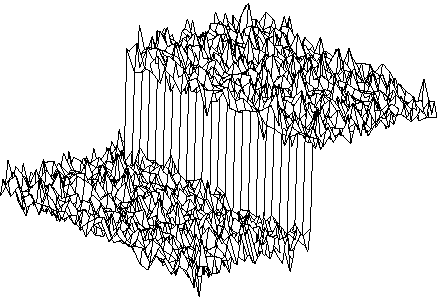(a)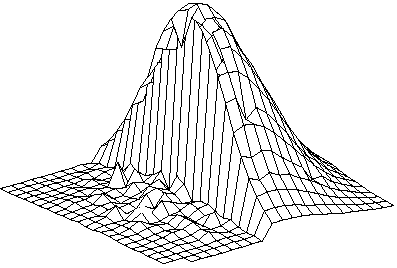(b)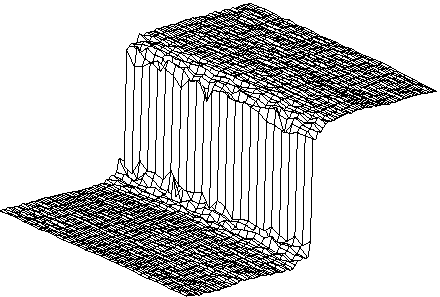(c)
Figure 1

当双边滤波器居中时，比如说，在边界亮边的一个像素上，相似性函数s对于同一边的像素采用接近于1的值，而对于黑色侧的像素接近于零。中心在灰度台阶右侧2个像素位置的23x23滤波器的相似函数如图1(a)所示。归一化项k（x）确保所有像素的权重合计为1。 结果，滤镜将中心处的亮像素替换为其附近的亮像素的平均值，并且基本上忽略了暗像素。 相反，当滤镜以暗像素为中心时，亮像素将被忽略。 因此，如图1（c）所示，得益于滤波器的域分量，在边界处获得了良好的滤波性能，并且由于范围分量，同时保留了清晰的边缘。

# The Gaussian Case

双边滤波器一个简单的重要的特例是平移不变的高斯滤波器，接近函数c和相似函数s是它们的参数之间的欧几里德距离的高斯函数。更特殊地是，c是径向对称的。

c(ξx)=e12(d(ξx)σd)2 c ( ξ − x ) = e − 1 2 ( d ( ξ − x ) σ d ) 2

d(ξx)=ξx d ( ξ − x ) = ‖ ξ − x ‖

是欧式距离。相似度函数s完全类似于接近函数c：
s(ξx)=e12(δ(f(ξ)f(x))σr)2 s ( ξ − x ) = e − 1 2 ( δ ( f ( ξ ) − f ( x ) ) σ r ) 2

δ(f(ξ)f(x))=|f(ξ)f(x)| δ ( f ( ξ ) − f ( x ) ) = | f ( ξ ) − f ( x ) |

是灰度级强度空间中距离的合适的度量。在标量情况下，这可能仅仅是像素差异的绝对差异，或者由于噪声随着图像灰度值的增加而增加，这是与灰度级的值有关的函数。正如这种形式的域滤波是平移不变的，上面介绍的高斯范围滤波对图像灰度级总体的附加变化不敏感。当然，范围滤波器也是平移不变的。

# Experiments with Black-and-White Images

图像2(a)和(b)显示了双边滤波器去除图像纹理的潜能。图2(b)说明的“图像简化”可以在不损失图像整体形状特征的情况下实现数据的压缩，这可以应用到图像传输，照片编辑和处理，图像检索。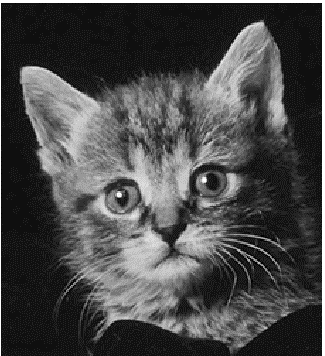(a)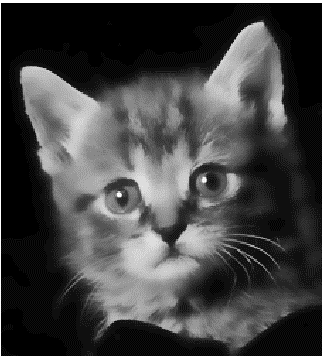(b)
Figure 2

对图像3(a)进行双边滤波，滤波器的参数 σd =3 σr =50，滤波结果如图3(b)所示。大多数细密的纹理已经被滤除掉了，但所有的轮廓和原始的图像中一样清晰。图像3(c)显示了图像3(a)的细节，图像3(d)显示了图像3(b)对应的细节部分。这2个图中的洋葱呈现相似的外观，细密的纹理已经被滤除了。然而，总体着色被保留下来，因为它完全位于域过滤器的范围内，并且几乎不受范围过滤器的影响。 此外，洋葱的边界被保留下来。(a)(b)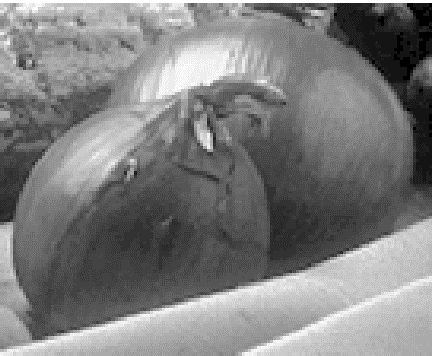(c)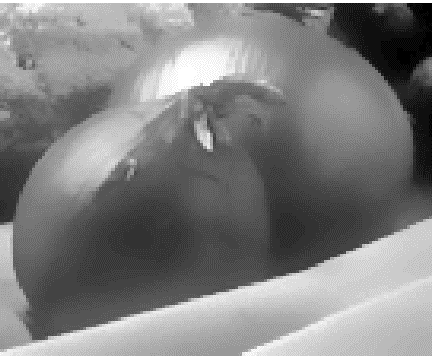(d)
Figure 3

# Experiments with Color Images

对于黑白图像，任何两个灰度级之间的强度值仍然是灰度值。 因此，当使用标准低通滤波器对黑白图像进行平滑处理时，会在边缘处产生中等灰度级，从而产生模糊的图像。对于彩色图像来说，由于任何两种颜色之间存在其他颜色，往往是截然不同的颜色，所以会产生额外的复杂性。 例如，在蓝色和红色之间有各种各样程度的粉红色和紫色。 因此，当跨越彩色边缘平滑时，可能产生干扰色带。 平滑后的图像不仅看起来模糊，还在物体周围展现出看起来奇怪的彩色光环。(a)(b)(c)(d)
Figure 4

图4（a）显示了一张对着蓝色天空的红色外套照片的细节。即使在这张没有模糊的照片中，也可以看到一条薄的粉红色紫色线条，这是由镜头模糊和像素平均相结合造成的。事实上，当投射回现场时，沿着边界的像素与红色外套和蓝色天空相交，所得到的颜色是粉红色，红色和蓝色的平均值。平滑时，突出了这种效果，如图4（b）中宽的而模糊的粉红色紫色区域所示。
为了解决这个困难，可以将边缘保留的平滑滤波分别应用于图像的红色，绿色和蓝色通道图像。但是，三个通道图像中边缘处的强度分布通常是不同的。如图4（c）所示，分别地平滑三个通道图像，会产生比原始色带更明显的粉红色和紫色带。然而，粉红色紫色带没有像图4（b）的标准模糊版本那样变宽。
双边过滤可以获得更好的结果。实际上，双边滤波器允许合适地组合三个色带，并测量组合空间中像素之间的光度距离。此外，通过在CIE-Lab色彩空间中使用欧几里得距离，可以使这种组合距离与感知的不相似性密切对应。该色彩空间基于大量由人类观察者进行的关于色彩匹配实验的心理物理学数据。在这个空间中，小的欧几里德距离被设计为，与“平均”颜色正常的人类观察者所经历的颜色差异的感知强烈相关。因此，从某种意义上说，在CIE-Lab色彩空间中执行的双边滤波是彩色图像最自然的滤波类型：只将知觉上相似的颜色一起取平均值，并且只保留感知上重要的边缘。图4（d）显示了由图4（a）中的图像双边平滑产生的图像。粉红色的带子大大缩小，并且不会出现无关的颜色。(a)(b)(c)
Figure 5

图5（c）显示了图5（a）中图像的双边滤波的五次迭代的结果。尽管单次迭代产生比原始图像更清晰的图像（图5（b）），并且对于大多数图像处理需求来说可能已足够，但多次迭代具有使图像中的颜色显着变平的效果，但没有模糊边缘。 生成的图像具有更小的颜色映射，并且在打印页面上显示时，双向过滤的效果更容易看到。 注意图5（c）的卡通状外观。 所有的阴影和边缘都被保留下来，但大部分阴影都没有了，滤波器不会引入“新”颜色。

展开全文双边滤波算法
• 双边滤波的matlab代码，压缩包内中包括试验所用的图片，试验所用的代码，由于是课程作业，所以代码亲测可用。滤波器
• 双边滤波器论文“Bilateral filtering for gray and color images”代码复现及其改进代码 matlab代码
• 可以实现，简洁易懂，根据论文所编。包括对灰度图像，彩色（RGB）图像进行双边滤波。既能平滑图像，用能保持边缘信息。
• 悲剧只能复制黏贴了双边滤波器是什么？双边滤波(Bilateral filter)是一种可以保边去噪的滤波器。之所以可以达到此去噪效果，是因为滤波器是由两个函数构成。一个函数是由几何空间距离决定滤波器系数。另一个由像素...

CSDN如何转载别人文章啊？！！！

悲剧只能复制黏贴了

双边滤波器是什么？

双边滤波(Bilateral filter)是一种可以保边去噪的滤波器。之所以可以达到此去噪效果，是因为滤波器是由两个函数构成。一个函数是由几何空间距离决定滤波器系数。另一个由像素差值决定滤波器系数。可以与其相比较的两个filter：高斯低通滤波器(http://en.wikipedia.org/wiki/Gaussian_filter)和α-截尾均值滤波器(去掉百分率为α的最小值和最大之后剩下像素的均值作为滤波器)，后文中将结合公式做详细介绍。

双边滤波器中，输出像素的值依赖于邻域像素的值的加权组合，权重系数w(i,j,k,l)取决于定义域核和值域核的乘积同时考虑了空间域与值域的差别，而Gaussian Filter和α均值滤波分别只考虑了空间域和值域差别。

=======================================================================

双边滤波器的实现(MATLAB)：function B = bfilter2(A,w,sigma)

% Douglas R. Lanman, Brown University, September 2006.

% dlanman@brown.edu, http://mesh.brown.edu/dlanman

具体请见function B = bfltGray(A,w,sigma_d,sigma_r)函数说明。

%简单地说:

%A为给定图像，归一化到[0,1]的矩阵

%W为双边滤波器(核)的边长/2

%定义域方差σd记为SIGMA(1),值域方差σr记为SIGMA(2)

%%%%%%%%%%%%%%%%%%%%%%%%%%%%%%%%%%%%%%%%%%%%%%%%%%%%%%%%%%%%%

% Pre-process input and select appropriate filter.

function B = bfilter2(A,w,sigma)

% Verify that the input image exists and is valid.

if~exist('A','var') || isempty(A)

error('Input image A is undefined or invalid.');

end

if~isfloat(A) || ~sum([1,3] == size(A,3)) || ...

min(A(:))  1

error(['Input image A must be a double precision ',...

'matrix of size NxMx1 or NxMx3 on the closed ',...

'interval [0,1].']);

end

% Verify bilateral filter window size.

if~exist('w','var') || isempty(w) || ...

numel(w) ~= 1 || w

w = 5;

end

w = ceil(w);

% Verify bilateral filter standard deviations.

if~exist('sigma','var') || isempty(sigma) || ...

numel(sigma) ~= 2 || sigma(1) <= 0 || sigma(2) <= 0

sigma = [3 0.1];

end

% Apply either grayscale or color bilateral filtering.

ifsize(A,3) == 1

B = bfltGray(A,w,sigma(1),sigma(2));

else

B = bfltColor(A,w,sigma(1),sigma(2));

end

%%%%%%%%%%%%%%%%%%%%%%%%%%%%%%%%%%%%%%%%%%%%%%%%%%%%%%%%%%%%%

% Implements bilateral filtering forgrayscale images.

function B = bfltGray(A,w,sigma_d,sigma_r)

% Pre-compute Gaussian distance weights.

[X,Y] = meshgrid(-w:w,-w:w);

%创建核距离矩阵，e.g.

%  [x,y]=meshgrid(-1:1,-1:1)

%

% x =

%

%     -1     0     1

%     -1     0     1

%     -1     0     1

%

%

% y =

%

%     -1    -1    -1

%      0     0     0

%      1     1     1

%计算定义域核

G = exp(-(X.^2+Y.^2)/(2*sigma_d^2));

% Create waitbar.

h = waitbar(0,'Applying bilateral filter...');

set(h,'Name','Bilateral Filter Progress');

% Apply bilateral filter.

%计算值域核H 并与定义域核G 乘积得到双边权重函数F

dim = size(A);

B = zeros(dim);

fori = 1:dim(1)

forj = 1:dim(2)

% Extract local region.

iMin = max(i-w,1);

iMax = min(i+w,dim(1));

jMin = max(j-w,1);

jMax = min(j+w,dim(2));

%定义当前核所作用的区域为(iMin:iMax,jMin:jMax)

I = A(iMin:iMax,jMin:jMax);%提取该区域的源图像值赋给I

% Compute Gaussian intensity weights.

H = exp(-(I-A(i,j)).^2/(2*sigma_r^2));

% Calculate bilateral filter response.

F = H.*G((iMin:iMax)-i+w+1,(jMin:jMax)-j+w+1);

B(i,j) = sum(F(:).*I(:))/sum(F(:));

end

waitbar(i/dim(1));

end

% Close waitbar.

close(h);

%%%%%%%%%%%%%%%%%%%%%%%%%%%%%%%%%%%%%%%%%%%%%%%%%%%%%%%%%%%%%

% Implements bilateral filter forcolor images.

function B = bfltColor(A,w,sigma_d,sigma_r)

% Convert input sRGB image to CIELab color space.

ifexist('applycform','file')

A = applycform(A,makecform('srgb2lab'));

else

A = colorspace('Lab

end

% Pre-compute Gaussian domain weights.

[X,Y] = meshgrid(-w:w,-w:w);

G = exp(-(X.^2+Y.^2)/(2*sigma_d^2));

% Rescale range variance (usingmaximum luminance).

sigma_r = 100*sigma_r;

% Create waitbar.

h = waitbar(0,'Applying bilateral filter...');

set(h,'Name','Bilateral Filter Progress');

% Apply bilateral filter.

dim = size(A);

B = zeros(dim);

fori = 1:dim(1)

forj = 1:dim(2)

% Extract local region.

iMin = max(i-w,1);

iMax = min(i+w,dim(1));

jMin = max(j-w,1);

jMax = min(j+w,dim(2));

I = A(iMin:iMax,jMin:jMax,:);

% Compute Gaussian range weights.

dL = I(:,:,1)-A(i,j,1);

da = I(:,:,2)-A(i,j,2);

db = I(:,:,3)-A(i,j,3);

H = exp(-(dL.^2+da.^2+db.^2)/(2*sigma_r^2));

% Calculate bilateral filter response.

F = H.*G((iMin:iMax)-i+w+1,(jMin:jMax)-j+w+1);

norm_F = sum(F(:));

B(i,j,1) = sum(sum(F.*I(:,:,1)))/norm_F;

B(i,j,2) = sum(sum(F.*I(:,:,2)))/norm_F;

B(i,j,3) = sum(sum(F.*I(:,:,3)))/norm_F;

end

waitbar(i/dim(1));

end

% Convert filtered image back to sRGB color space.

ifexist('applycform','file')

B = applycform(B,makecform('lab2srgb'));

else

B = colorspace('RGB

end

% Close waitbar.

close(h);

调用方法：

I=double(I)/255;

w     = 5;       % bilateral filter half-width

sigma = [3 0.1]; % bilateral filter standard deviations

I1=bfilter2(I,w,sigma);

subplot(1,2,1);

imshow(I);

subplot(1,2,2);

imshow(I1)

实验结果：参考资料：

1.《Computer Vision Algorithms and Applications》

5. http://mesh.brown.edu/dlanman

展开全文• 这是 3D 图像快速双边滤波的实现。 该过滤器在保留边缘的同时平滑图像，但在其最直接的实现中对计算的要求非常高，尤其是对于大型 3D 图像。 快速双边滤波（S. Paris 和 F. Durand，麻省理工学院 ）是一种近似...matlab
•滤波器
• %% Bilateral双边滤波器 clc,clear,close all % 清理命令区、清理工作区、关闭显示图形 warning off % 消除警告 feature jit off % 加速代码运行 [filename ,pathname]=… uigetfile({‘.bmp’;'.tif’;‘*.jpg’;},...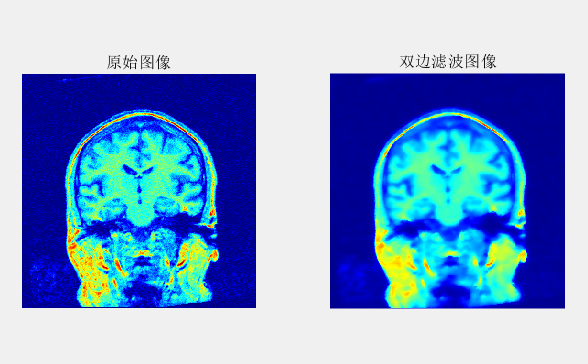%% Bilateral双边滤波器
clc,clear,close all  % 清理命令区、清理工作区、关闭显示图形
warning off       % 消除警告
feature jit off      % 加速代码运行
[filename ,pathname]=...
uigetfile({'*.bmp';'*.tif';'*.jpg';},'选择图片'); %选择图片路径
str=[pathname filename]; % 合成路径+文件名
im = imnoise(im,'gaussian',0,1e-3); % 原图像 + 白噪声

figure,
subplot(121),imshow(im);title('原始图像')
colormap(jet)  % 颜色
[im1, PSNR] = bif_filter(im,3,0.2);
subplot(122),imshow(im1);title('双边滤波图像')
colormap(jet)  % 颜色

function [out, psn]=bif_filter(im,sigd,sigr)
% bilateral filter双边滤波器
% 函数输入：
%           im    输入的图像
%           sigd  空间内核的时域参数
%           sigr  内核参数强度变化范围
% 函数输出：
%          out  滤波图像 = output imagespatial kernel

w=(2*sigd)+1;
% sigr=(n*100)^2/(.003*(sigd^2));  % 自适应R值，n为高斯噪声强度,n=0.001

% 高斯滤波器
gw=zeros(w,w);       % 高斯权值矩阵初始化
c=ceil(w/2);         % 向前取整
c=[c c];             % 中心元素位置

for i=1:w
for j=1:w
q=[i,j]; % 记录相连像素位置标识位
gw(i,j)=norm(c-q); % 欧氏距离
end
end

Gwd=(exp(-(gw.^2)/(2*(sigd^2)))); % 高斯函数

% A = [1 2; 3 4];
% B =
%      1     2     2     2
%      3     4     4     4
%      3     4     4     4
%      3     4     4     4
%      3     4     4     4

[row clm]=size(proci);    % Size of image
if ~isa(proci,'double')
proci = double(proci)/255;   % 转换为double类型
end

K=sigd;
L=[-K:K];
c=K+1;   % 中心元素位置
iter=length(L); % 迭代次数
ind=1;

for r=(1+K):(row-K)          % 行
for s=(1+K):(clm-K)      % 列
for i=1:iter     % 窗口大小 行
for j=1:iter % 窗口大小 列
win(i,j)=proci((r+L(i)),(s+L(j))); % 获取窗口
end
end
I=win; % 灰度矩阵
win=win(c,c)-win; % 相对中心点处的强度差异，中心点为参考灰度值
win=sqrt(win.^2); % 保证win中的每一个元素为正
Gwi=exp(-(win.^2)/(2*(sigr^2))); % 高斯函数
weights=(Gwi.*Gwd)/sum(sum(Gwi.*Gwd)); % 高斯权值
Ii=sum(sum(weights.*I));               % 得到当前双边滤波值
proci(r,s)=Ii;                         % 替换当前灰度值
win=[];
end
end

% 移除边界扩展值
out=im2uint8(proci);   % 类型转换

%% 滤波重建后，图像峰值信噪比计算
if ~isa(out,'double')
dimg = double(out)/255;   % 转换为double类型
end
psn = PSN(im,dimg); % PSNR,峰值信噪比

end

% 移除边界扩展值
% 函数输入：
%         R    输入的图像矩阵
%         K    窗口大小(2*K + 1)
% 函数输出：
%         x    移除边界扩展值后的原图像矩阵
for i=1:K
R(1,:)=[];
R(:,1)=[];
[ro cl]= size(R);
R(ro,:)=[];
R(:,cl)=[];;
end
x=R;
end

function [out]=PSN(orgimg,mimg)
% 峰值信噪比计算

orgimg =im2double(orgimg);
mimg   =im2double(mimg);
Mse=sum(sum((orgimg-mimg).^2))/(numel(orgimg)); % Mean square Error均方差
out=10*log10(1/Mse);
end

展开全文matlab
• 双边滤波器的函数实现。可自行选择文件路径，用双边滤波法进行去噪处理，并将结果显示在屏幕上。
• 提出了一种”基于小波变换的多尺度自适应双边滤波器“算法。 其对应的算法流程如下所示： 下面，我们从理论上限介绍一下这里所采用的改进后的算法。 第一：多尺度图像的自适应双边滤波 这个部分，是...
• 原创文章 346获赞 632访问量 51万+ 关注 他的留言板 展开阅读全文 作者：颹蕭蕭
• 相比较而言，bilateral filter双边滤波器可以很好的边缘保护，即可以在去噪的同时，保护图像的边缘特性。双边滤波(Bilateral filter)是一种非线性的滤波方法，是结合图像的空间邻近度和像素值相似度的一种折衷处理，...c语言双边滤波算法
• 这是一个matlab程序,用双边滤波器实现了图像内插....